2022: SklogWiki celebrates 15 years on-line

# Ramp model

(diff) ← Older revision | Latest revision (diff) | Newer revision → (diff)

The ramp model, proposed by Jagla  and sometimes known as the Jagla model, is described by: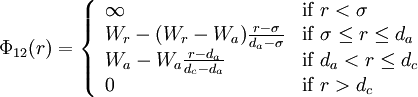$\Phi_{12}(r) = \left\{ \begin{array}{ll} \infty & {\rm if} \; r < \sigma \\ W_r - (W_r-W_a) \frac{r-\sigma}{d_a-\sigma} & {\rm if} \; \sigma \leq r \leq d_a \\ W_a - W_a \frac{r-d_a}{d_c-d_a} & {\rm if} \; d_a < r \leq d_c \\ 0 & {\rm if} \; r > d_c \end{array} \right.$

where$\Phi_{12}(r)$ is the intermolecular pair potential,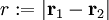$r := |\mathbf{r}_1 - \mathbf{r}_2|$,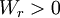$W_r > 0$ and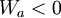$W_a < 0$.

Graphically, one has:

where the red line represents an attractive implementation of the model, and the green line a repulsive implementation.

## Critical points

For the particular case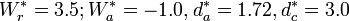$W_r^*=3.5; W_a^*=-1.0, d_a^*=1.72, d_c^*=3.0$, the liquid-vapour critical point is located at :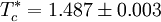$T_c^* = 1.487 \pm 0.003$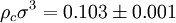$\rho_c \sigma^3 = 0.103 \pm 0.001$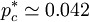$p_c^* \simeq 0.042$

and the liquid-liquid critical point: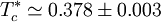$T_c^* \simeq 0.378 \pm 0.003$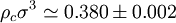$\rho_c \sigma^3 \simeq 0.380 \pm 0.002$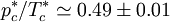$p_c^*/T_c^* \simeq 0.49 \pm 0.01$

While this liquid-liquid critical point was long held to be in the stable region of the phase diagram, a high density double-network structure was found to be thermodynamically more stable than the high-density liquid under any conditions.:

## Repulsive Ramp Model

In the repulsive ramp case, where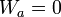$W_a = 0$, neither liquid-vapor nor liquid-liquid stable equilibria occur . However, for this model a low density crystalline phase has been found. This solid phase presents re-entrant melting, i.e. this solid melts into the fluid phase as the pressure is increased.

#### Lattice gas version

Recently, similar behaviour has been found in a three-dimensional Repulsive Ramp Lattice Gas model  The system is defined on a simple cubic lattice. The interaction is that of a lattice hard sphere model with exclusion of nearest neighbours of occupied positions plus a repulsive interaction with next-to-nearest neighbours. The total potential energy of the system is then given by: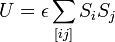$U = \epsilon \sum_{[ij]} S_i S_j$

where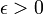$\epsilon > 0$ ;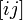$[ij]$ refers to all the pairs of sites that are second neighbors, and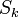$S_k$ indicates the occupation of site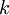$k$ (0 indicates an empty site, 1 indicates an occupied site).